Good habits: aggregate and optimize
Share
Explore
Filtering data in place
Approach
Using the Filter() formula in every column.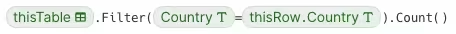The formula above will go through every row of the table, and for each row, it will compare the Country value to that of every other row in the table.
The table contains 1,000 rows with multiple data points for 185 countries. If the first row contains sales data from Afghanistan, every row in that table in the table will be evaluated to see if it contains the word “Afghanistan”. In this table of 1,000 rows, the first row will need to run this comparison 1,000 times.
This calculation repeats for every single row in the table. We can calculate our estimate of operations.
1,000 calculations for one row multiplied by 1,000 rows equals 1,000,000 calculations.
In Big O Notation, this is O(N^2).
This also means that if the same country shows up in multiple rows, we still run the calculations every time.
Let’s say that you now want to filter the table based on another data point, by country and sales made after a certain date. We need to repeat the filter part of the formula from above and add the date criteria.
With the total count in one column and the more specific count in another column, we run through 1 million calculations for each column. So every additional data point we ask for become equally as expensive as the first.
We see this approach most often when people are moving over to Coda from the world of spreadsheets. Everything is in one table, and when more data is needed, it’s familiar to just add more columns.
That thought process makes sense, but Coda offers some tools to give you super powers when it comes to working with data. We’ll explore these features in and .
Strategy one: sales report =
1000
rows
Search
1
Count of Sales Orders per Country
Calculations: 1,000,000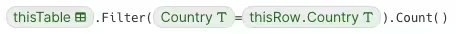2
Total Units Sold per Country
Calculations: 1,000,000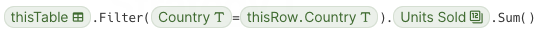3
Total Profit per Country
Calculations: 1,000,000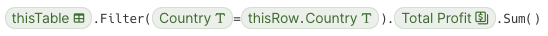4
Total Cost per Country
Calculations: 1,000,000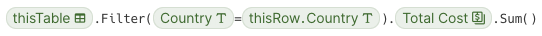5
Most Recent Ship Date per Country
Calculations: 1,000,000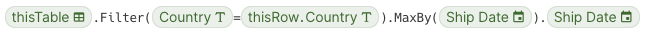There are no rows in this table
Total Calculations for five data points: 5,000,000
We end up getting the answers we’re looking for, but they are very repetitive. Every row listing the same country is also going to list the same aggregate data points as the others.
If we decide we want to show this data in a chart, we start having to play games to find unique values only.
As part of Coda’s ongoing performance work, our engineers have implemented various ways for us to “pre-process” data when similar patterns are found across a doc, so the impact you see isn’t where the estimates above would suggest. But the best building practices on the next few pages will reveal more benefits beyond performance making it worth while to strive for good building habits.
Let’s check out strategy two
Strategy one: sales report
0